Solving for Eigenvalues of Simultaneous

Linear First Order ODEs

Given: A set of linear 1st Order ODEs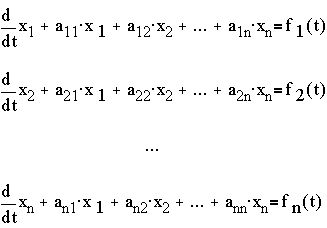Want to Find:  the eigenvalues of the system
To discuss the two methods, we will restrict the system to n=2. The general method would just follow similar process.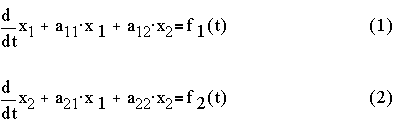Method 1: By Substitution

Step 1: take derivative of (1),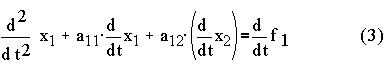Step 2: substitute (2) into (3),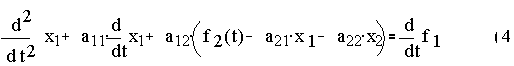Step 3: From (1), get x2 in terms of x1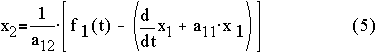Step 4: Substitute (5) into (4) and rearrange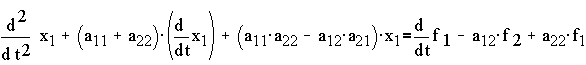Thus the characteristic equation will be given by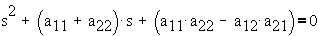Note: If we had tried to find a second order differential equation in terms of x2 using the same procedure of substitution, we will obtain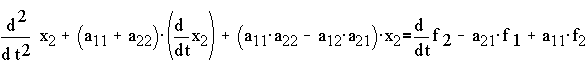which will yield the same characteristic equation. Thus there is really just one characteristic equation for the system.

The eigenvalues will just be the roots of the characteristic equation.

Example:Then the characteristic equation and the eigenvalues are:The system is unstable.

Method 2: By Operator Matrices Step 1: Replace the derivatives by differential operators, D = d/dt,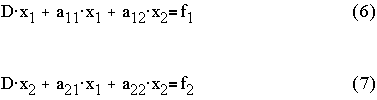Step 2: Extract the matrix operator,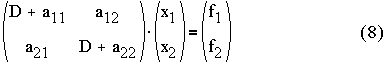Step 2: Use Cramers rule,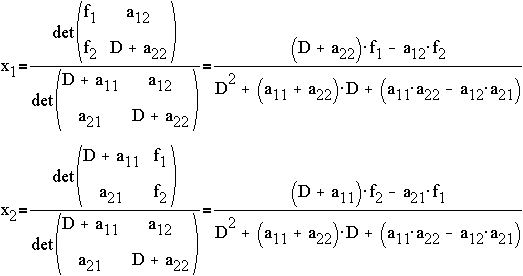or,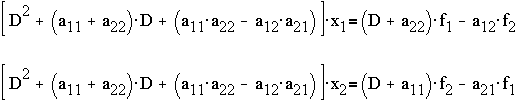which is the same result as Method 1 when D is replaced back by d/dt.

Generalization: The characteristic equation for a set of n linear first order ODEscan be obtained as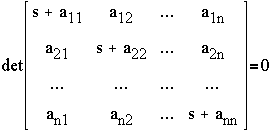Example:

For the system given by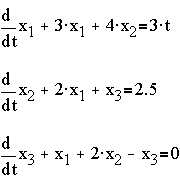The characteristic equation and the eigenvalues are given by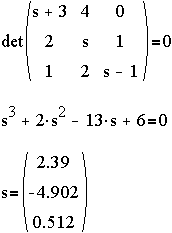The system is unstable.

This page is maintained by Tomas B. Co (tbco@mtu.edu). Last revised 12/10/1999.

Tomas B. Co
Associate Professor
Department of Chemical Engineering
Michigan Technological University
1400 Townsend Avenue
Houghton, MI 49931-1295